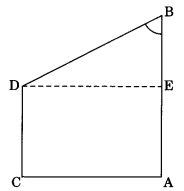Check the below Online Education NCERT MCQ Questions for Class 10 Maths Chapter 8 Introduction to Trigonometry with Answers Pdf free download. MCQ Questions for Class 10 Maths with Answers were prepared based on the latest exam pattern. We have provided Introduction to Trigonometry Class 10 Maths MCQs Questions with Answers to help students understand the concept very well. https://ncertmcq.com/mcq-questions-for-class-10-maths-with-answers/

Students can also refer to NCERT Solutions for Class 10 Maths Chapter 8 Introduction to Trigonometry for better exam preparation and score more marks.

## Introduction to Trigonometry Class 10 MCQs Questions with Answers

Trigonometry Class 10 MCQ Question 1.
Given that sin θ = $$\frac{a}{b}$$ then cos θ is equal to
(a) $$\frac{b}{\sqrt{b^2-a^2}}$$
(b) $$\frac{b}{a}$$
(c) $$\frac{\sqrt{b^2-a^2}}{b}$$
(d) $$\frac{a}{\sqrt{b^2-a^2}}$$

Answer: (c) $$\frac{\sqrt{b^2-a^2}}{b}$$

Trigonometry MCQ Class 10 Question 2.
Given that sin α = $$\frac{1}{2}$$ and cos β = $$\frac{1}{2}$$, then the value of (α + β) is
(a) 0°
(b) 30°
(c) 60°
(d) 90°Introduction To Trigonometry Class 10 MCQ Question 3.
If tan θ = 3, then $$\frac{4sin θ-cos θ }{4sin θ+cos θ}$$ is equal to
(a) $$\frac{2}{3}$$
(b) $$\frac{1}{3}$$
(c) $$\frac{1}{2}$$
(d) $$\frac{3}{4}$$

Answer: (c) $$\frac{1}{2}$$

Class 10 Trigonometry MCQ Question 4.
sin (45° + θ) – cos (45° – θ) is equal to
(a) 2 cos θ
(b) 0
(c) 2 sin θ
(d) 1

MCQ Of Trigonometry Class 10 Question 5.
If √2 sin (60° – α) = 1 then α is
(a) 45°
(b) 15°
(c) 60°
(d) 30°

MCQ Trigonometry Class 10 Question 6.
The value of sin² 30° – cos² 30° is
(a) –$$\frac{1}{2}$$
(b) $$\frac{√3}{2}$$
(c) $$\frac{3}{2}$$
(d) –$$\frac{2}{3}$$

Answer: (a) –$$\frac{1}{2}$$

Class 10 Maths Chapter 8 MCQ Question 7.
The maximum value of $$\frac{1}{cosec α}$$ is
(a) 0
(b) 1
(c) $$\frac{√3}{2}$$
(d) –$$\frac{1}{√2}$$

MCQ On Trigonometry For Class 10 Pdf Question 8.
If cos (40° + A) = sin 30°, then value of A is
(a) 30°
(b) 40°
(c) 60°
(d) 20°

Class 10 Maths Trigonometry MCQs Question 9.
If cosec θ – cot θ = $$\frac{1}{3}$$, the value of (cosec θ + cot θ) is
(a) 1
(b) 2
(c) 3
(d) 4

Trigonometry Class 10 MCQ With Answers Question 10.
In the given figure, if AB = 14 cm, BD = 10 cm and DC = 8 cm, then the value of tan B is(a) $$\frac{4}{3}$$
(b) $$\frac{14}{3}$$
(c) $$\frac{5}{3}$$
(d) $$\frac{13}{3}$$

Answer: (a) $$\frac{4}{3}$$

Class 10 Introduction To Trigonometry MCQ Question 11.
$$\frac{1+tan^2 A}{1+cot^2 A}$$ is equal to
(a) sec² A
(b) -1
(c) cot² A
(d) tan² A

Ch 8 Maths Class 10 MCQ Question 12.
If cos A + cos² A = 1, then sin² A + sin4 A is equal to
(a) -1
(b) 0
(c) 1
(d) None of these

Class 10 Maths Ch 8 MCQ Question 13.
If sin θ + sin² θ = 1 then cos² θ + cos4 θ is equal
(a) -1
(b) 1
(c) 0
(d) None of these

MCQ On Trigonometry Class 10 Question 14.
2(sin6 θ + cos6 θ) – 3(sin4 θ + cos4 θ) is equal to
(a) 0
(b) 6
(c) -1
(d) None of these

Class 10 Trigonometry MCQ Questions Question 15.
If cos (81 + θ)° = sin($$\frac{k}{3}$$ – θ)° where θ is an acute angle, then the value of k is
(a) 18°
(b) 27°
(c) 9°
(d) 81°

Question 16.
3 sin² 20° – 2 tan² 45° + 3 sin² 70° is equal to
(a) 0
(b) 1
(c) 2
(d) -1

Question 17.
If sin 2A = $$\frac{1}{2}$$ tan² 45° where A is an acute angle, then the value of A is
(a) 60°
(b) 45°
(c) 30°
(d) 15°

Question 18.
$$\frac{sin θ}{1 + cos θ}$$ is
(a) $$\frac{cos θ}{1 – sin θ}$$
(b) $$\frac{1 – sin θ}{sin θ}$$
(c) $$\frac{1 – sin θ}{cos θ}$$
(d) $$\frac{1 – cos θ}{sin θ}$$

Answer: (d) $$\frac{1 – cos θ}{sin θ}$$

Question 19.
If x sin (90° – θ) cot (90° – θ) = cos (90° – θ), then x is equal to
(a) 0
(b) 1
(c) -1
(d) 2Question 20.
If A + B = 90°, cot B = $$\frac{3}{4}$$ then tan A is equal to:
(a) $$\frac{5}{3}$$
(b) $$\frac{1}{3}$$
(c) $$\frac{3}{4}$$
(d) $$\frac{1}{4}$$

Answer: (c) $$\frac{3}{4}$$